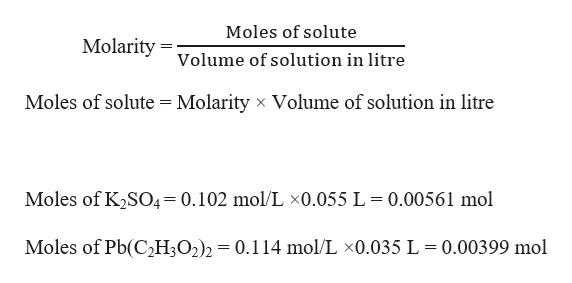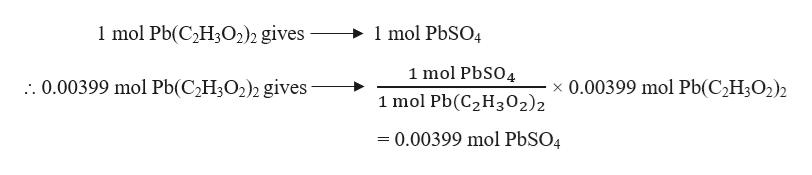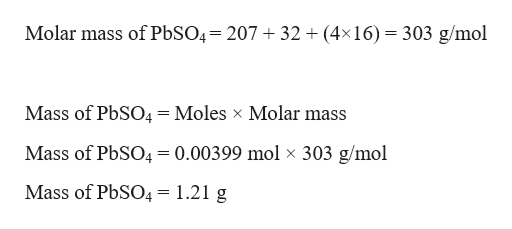A 55.0mL sample of 0.102M potassium sulfate solution is mixed with 35.0mL of a 0.114M lead(II) acetate solution and the precipitation reaction shown here occurs:K2SO4(aq)+Pb(C2H3O2)2(aq)=2KC2H3O2(aq)+PbSO4(s).Determine the theoretical yield of PbSO4 in grams.

Question

A 55.0mL sample of 0.102M potassium sulfate solution is mixed with 35.0mL of a 0.114M lead(II) acetate solution and the precipitation reaction shown here occurs:

K2SO4(aq)+Pb(C2H3O2)2(aq)=2KC2H3O2(aq)+PbSO4(s).

Determine the theoretical yield of PbSO4 in grams.

Step 1

Given,

Molarity of potassium sulfate solution = 0.102 M = 0.102 mol/L

Volume of potassium sulfate solution = 55.0 mL = 0.055 L     (1mL = 0.001 L)

Molarity of lead(II) acetate solution = 0.114 M = 0.114 mol/L

Volume of lead(II) acetate solution = 35.0 mL = 0.035 L     (1mL = 0.001 L)

Moles of potassium sulfate, K2SO4 and lead(II) acetate, Pb(C2H3O2)2 can be calculated as:help_outlineImage TranscriptioncloseMoles of solute Molarity= volume of solution in litre Moles of solute Molarity x Volume of solution in litre Moles of K2SO4=0.102 mol/L x0.055 L = 0.00561 mol Moles of Pb(C2H3O2)2 = 0.114 mol/L x0.035 L 0.00399 mol fullscreen
Step 2

The balanced reaction is given as:

K2SO4 (aq) + Pb(C2H3O2)2 (aq) → 2 KC2H3O2 (aq) + PbSO4 (s)

Stoichiometric molar ratio of K2SO4  : Pb(C2H3O2)2  = 1 : 1

Actual molar ratio of K2SO4 : Pb(C2H3O2)2  = 0.00561 : 0.00399 = 1.4 : 1

Therefore, K2SO4 is in excess, so the limiting reagent is Pb(C2H3O2)2

Theoretical mass of PbSO4 produced can be calculated as:help_outlineImage Transcriptionclose1 mol PbSO4 1 mol Pb(C2H302)2 gives 1 mol PbSO4 . 0.00399 mol Pb(C2H3O2)2 gives x 0.00399 mol Pb(C2H302)2 1 mol Pb(C2H302)2 - 0.00399 mol PbSO4 fullscreen
Step 3

Mass of PbSO4 produced can be...help_outlineImage TranscriptioncloseMolar mass of P6SO4= 207 32 (4x16) 303 g/mol Mass of PbSO4=Moles x Molar mass Mass of PbSO4= 0.00399 mol x 303 g/mol Mass of PbSO4 = 1.21 g fullscreen

Want to see the full answer?

See Solution

Want to see this answer and more?

Our solutions are written by experts, many with advanced degrees, and available 24/7

See Solution
Tagged in

General Chemistry